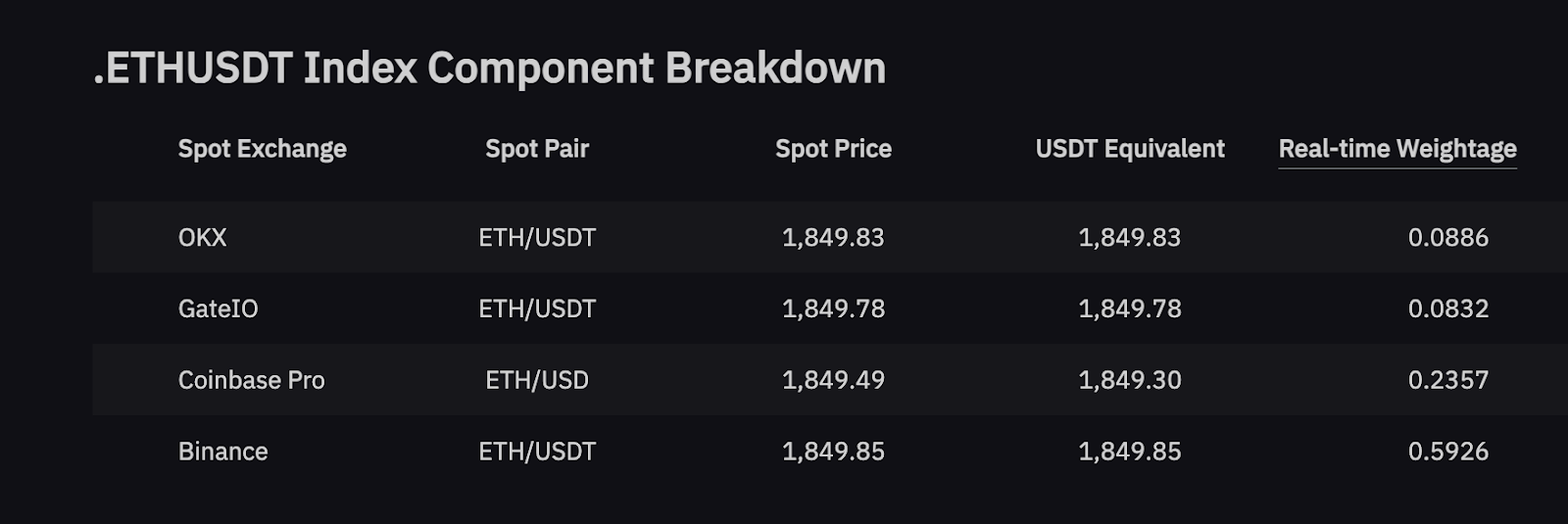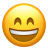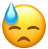# Index Price Calculation2022-07-20 11:06:11

The index price is the sum of the prices of the top six (6) Spot trading pairs on the major Spot exchanges by trading volume, multiplied by the respective weights of the Spot trading pairs (The symbol — .XXXUSDT, XXX — is represented by the respective coin's abbreviations, such as BTC, ETH, XRP or EOS.). Index Price can be obtained from the underlying data pages for the Inverse Contract and USDT Perpetual Contract, respectively.

The index price depends on three (3) variables: Spot Price, USDT-Paired Equivalent and Real-time WeightSpot Price

This figure represents the current live price quoted directly from the respective Spot exchanges for the underlying coin asset.

USDT-Paired Equivalent

This data represents the price of Spot trading pairs converted into USDT trading pairs, based on the current quote.

Example

Let's consider a scenario where the ETHUSDT index includes a component from Exchange A using the trading pair ETH/BTC, with a current quote of 0.1. If the current BTC/USDT price on the Bybit stands at \$20,000. In this case, the USDT-paired equivalent value is \$2,000 based on the following calculation:

Current Quote × BTC/USDT = 0.1 × 20,000

Real-time Weight

The Index Price is calculated by summing the weighted prices of Spot trading pairs from top global Spot exchanges. The weight, known as Trade_WtO, is based on the four-hour trading volumes of the six (6) leading Spot trading pairs. This weight is then applied to the current quote price to determine its impact on the overall Index Price. For clarity, we'll refer to the platforms as A, B, C, D, E, and F in the following examples.

Index Price Calculation

The calculation formula is as follows:

Index Price = (Spot Price_Symbol A × Trade_WtO_Symbol A) + (Spot Price_Symbol B × Trade_WtO_Symbol B) + (Spot Price_Symbol C × Trade_WtO_Symbol C) + (Spot Price_Symbol D × Trade_WtO_Symbol D) + (Spot Price_Symbol E × Trade_WtO_Symbol E) + (Spot Price_Symbol F × Trade_WtO_Symbol F)

To ensure stability in the index price during market volatility, we've introduced a price protection mechanism:

1. If the Spot price of any component trading pair diverges by more than 5% from the median of all Spot price sources, it will be temporarily excluded from the index price calculation. This exclusion lasts until its price lies within 3% of all Spot prices' midpoint for a continuous 5-minute period. However, this rule doesn't apply to certain designated trading pairs.

2. If two (2) or more Spot prices of the component trading pairs deviate more than 5% from the median price, the index price will remain as the trading volume—weighted average of all price resources.

3. To remove trading pairs that are suffering from liquidity issues or experiencing a service disruption, if no Spot trading pair has been traded on the exchange for more than 15 minutes, the trading pair will be excluded from the index price calculation. Once trading activities resume, they will once again be entered into the calculation.

4. If the Spot price experiences a delay of more than 5 seconds compared to the real-time transaction, that specific trading pair will be temporarily excluded from the calculation of the index price. Once the timing discrepancy is resolved, the trading pair will be reintegrated into the index price calculation.

5. In extreme market conditions or abnormal currency pair price fluctuations, Bybit reserves the right to adjust the price source or weight without prior notification.

Example

Let’s assume that the BTC Spot prices and trading volume weights for six (6) trading pairs are as follows:

 Spot Exchange Trading Pair Spot Price Weight A BTC/USDT \$20,046 20% B BTC/USDC \$20,048 15% C BTC/USDT \$20,056 20% D BTC/USDT \$20,058 15% E BTC/USDT \$20,060 15% F BTC/USDT \$20,051 15%

The .BTCUSDT index price is \$20,052.95 based on the following calculation:

Index Price = (\$20,046 × 20%) + (\$20,048 × 15%) + (\$20,056 × 20%) + (\$20,058 × 15%) + (\$20,060 × 15%) + (\$20,051 × 15%)YesNo